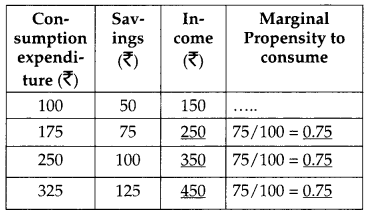## CBSE Previous Year Question Papers Class 12 Economics 2013 Delhi

Time allowed : 3 hours
Maximum marks: 100

** Answer is not given due to change in the present syllabus

### CBSE Previous Year Question Papers Class 12 Economics 2013 Delhi Set – I

Section – A

Question 1.
Give two examples of fixed costs. 

Question 2.
Define marginal cost. 
Marginal cost may be defined as the change in total cost that takes place by producing an additional unit.

Question 3.
When is the demand for a good said to be inelastic ? 
The demand for a good is inelastic when the percentage change in the demand of a product is less than the percentage change in the price of the good.

Question 4.
Give the meaning of market demand. 
Market demand is the sum total demand of all the buyers of a good at a point of time at a given price.

Question 5.
Under which mai……Fig…… a firm’s marginal revenue is always equal to price. 
Under perfect competition marginal revenue is always equal to price.

Question 6.
Explain the difference between an inferior good and a normal good. 
Normal Good
1. A normal good is one whose demand increases with an increase in the money income of the consumer.
2. Normal goods have positive income effect, e.g. if a consumer buys more of milk for his family as his income rises, then milk will be called a normal good as shown in Diagram 1.Inferior Good
1. An inferior good is one whose demand falls with a rise in income of the consumer because he can now afford to buy a normal (superior) good.
2. Inferior goods have negative income effect; e.g. if a consumer reduces the consumption of toned milk when his income rises, then toned milk is an inferior good for that consumer, as shown in figure.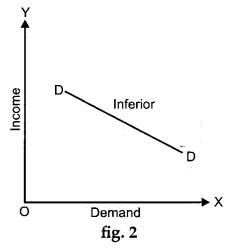Question 7.
Explain the law of diminishing marginal utility with the help of a total utility schedule. 
OR
Explain the condition of consumer’s equilibrium with the help of utility analysis. 
Law of Diminishing Marginal Utility states that as a consumer consumes more and more units of a commodity at succession, then the Marginal Utility derived from the consumption of each additional unit of the commodity falls.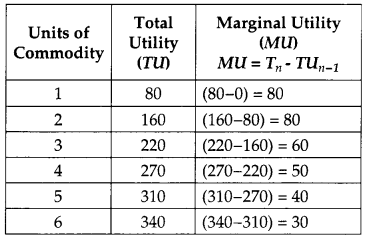From the above schedule, it can be observed that for two unit of consumption, marginal utility is 80. For the third unit, the marginal utility falls to 60. For the fourth unit, the marginal utility further falls to 50 and so on. Thus, as more and more units of a commodity are consumed, the marginal utility derived from the consumption of each additional unit falls.
OR
Consumer’s equilibrium will be attained at a point where marginal utility of a commodity is equal to its price. However, MU is expressed in terms of utils and price is expressed in money form. Therefore, equality of MU in utils and price cannot be the basis of consumer’s equilibrium. Hence, marginal utility also needs to be expressed in money form.

Marginal utility in money form can be obtained by dividing it (MU) by marginal utility of one rupee. Marginal utility of 1 is the extra utility when an additional rupee is spent on other available goods in general. Suppose that for an additional rupee we get two units of some other commodity, then marginal utility of rupee is 2 (utils). Knowing marginal utility (MU) of a commodity and marginal utility of a rupee, we can find out marginal utility of a commodity in money terms in the following way :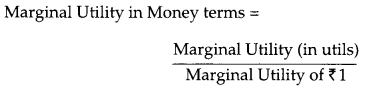Question 8.
When the price of a good rises from ₹ 20 per unit to ₹ 30 per unit, the revenue of the firm producing this good rises from ₹ 100 to ₹ 300. Calculate the price elasticity of supply. 
Price, P = ₹ 20, TR = ₹ 100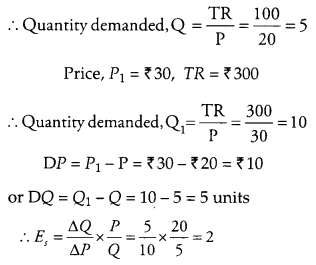Question 9.
Complete the following table :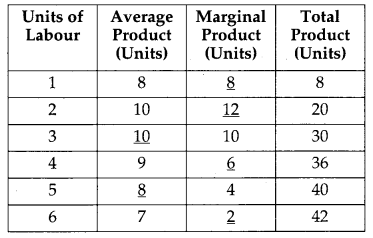Question 10.
Explain “large number of buyers and sellers” feature of a perfectly competitive market. 
There are very large number of buyers in perfect competition. No individual buyer can influence the market price because of its large number. Every buyer buys only a fraction from the total sale of the product.

Buyers like firms have to accept the price which is fixed by the supply and demand of the whole industry. Buyers can buy any quantity of product at this price. Similarly sellers are also very large in number having very small share in the market. Sellers cannot influrence the market price and can sell any quantity on the prevailing price. That is why firms are called price-takers in perfectly competitive industry.

Question 11.
Production in an economy is below its potential due to unemployment. Goverment starts employment generation schemes. Explain its effect using production possibilities curve. 
Unemployment in economy leads to underutilization of resources which compels an economy to remain on less efficient PPC. Hence the AD should be raised to increase employment. So the government should start some schemes through which can be generated. As a result the point of actual operation will move to or move nearer to the situation where there is a no unemployment. We can see in the diagram, P is the point of operation due to unemployment. The point of operation will move on to the right side of Production Possibility curve when the new employment schemes will be introduced. The point of operation shifts from point P to point M or T or any other point on the production possibility curve AB.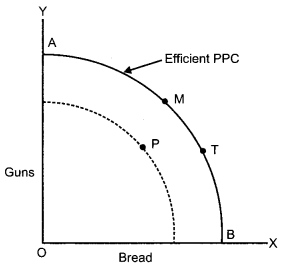Question 12.
Explain the conditions of producer’s equilibrium with the help of a numerical example. 
Producer’s equilibrium refers to a combination of price and quantity of output which yields the producer the maximum profit. For achieving this combination two conditions need to be fulfilled.

(i) The difference between total cost and total revenue must be the maximum.
(ii) At the point where, the difference between TC and TR is maximum, MR and MC should also be equal. Any departure from this position will not ensure maximum profit.

In the Table, the difference between TR and TC is maximum at 3 units as well as at 4 units but the point of equilibrium is 4 units because at this point MC = MR. & MC must rise.
These two conditions can be explained with the help of a table given below :
Equilibrium of a Producer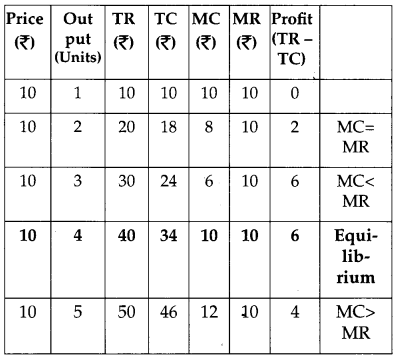Question 13.
The price elasticity of demand for a good is -0.4. If its price increase by 5 percent, by what percentage will its demand fall? Calculate.-2 = Percentage change in quantity demanded
∴ Quantity decreases by 2% or Demand falls by 2%

Question 14.
Giving reasons, state whether the following statements are true or false : 
(i) A monopolist can sell any quantity he likes at a price.
(ii) When equilibrium price of a good is less than its market price, there will be competition among the sellers.
(i) The statement is false. The price is fixed at a point where marginal cost is equal to marginal revenue. If a monopolist fixes a price less or more than the price fixed at equilibrium point, the quantity will be more or less than the equilibrium quantity but not the quantity that a monopolist would like to sell.

(ii) The statement is true. In this situation, market price is higher than the equilibrium price. As a result of which supply will be more than demand. As such there will be competition among the sellers. In other words, sellers would like to sell their entire quantity to meet the demand which (supply) is much more than the demand.

Question 15.
Explain the Law of Variable Proportions with the help of total product and marginal product curves. 
The law of variable proportions explains the relationship between inputs and outputs in the short run. In the short run, some factors of production (inputs) are fixed and other factors input are variables. The quantity of output can be increased by increasing the use of variable input.

As more and more units of variable input are employed, the proportion between the fixed and variable factors keeps on changing. The output passes through three phases.
These three phases are identified with respect to the marginal product.

Phase I: TP increases at an increasing rate. In The first phase of production the marginal product rises and reaches its highest point. This is the phase of Increasing Returns to a factor and during this phase, total product increases at an increasing rate.

Phase II: TP increases at a diminishing rate. In this phase, Marginal Product is declining but is positive. In this phase total product increases but at a diminishing rate. This phase ends when Marginal Product is zero and Total Product is at its maximum level. A producer always operates in this stage.

Phase III: TP is falling. In phase of production, Marginal Product is and negative. Here total product starts falling.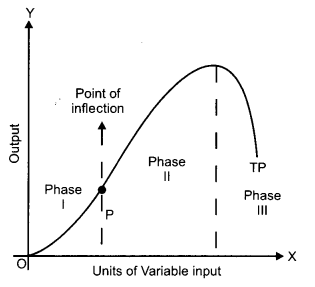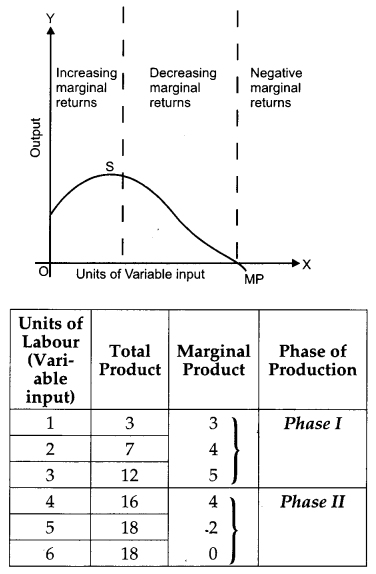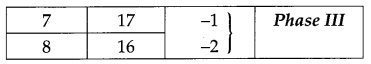These phases are shown graphically in diagram. The reasons for the Operation of the Law are :
1. Optimum combination of factors : The phase of increasing marginal product is due to optimum combination of factors that is required for any given technology, therefore fixed factors get better utilized.

2. However, when more and more units of variable factors are employed to a fixed factor, the fixed factor cannot absorb it and there is overcrowding of variable factors due to which the marginal product falls and becomes negative. This is the phase of diminishing marginal product.

Question 16.
Explain consumer’s equilibrium with the help of Indifference Curve Analysis. 
OR
Explain the relationship between
(i) Prices of other goods and demand for the given good.
(ii) Income of the buyers and demand for a good.
Consumer’s equilibrium refers to the optimum combination of the two goods which a consumer can afford (given his income and price of two commodities) and this combination gives him maximum satisfaction that he possibly can get.According to indifference curve analysis, consumer’s equilibrium is established at a point where budget line is tangent to the highest attainable indifference curve. At this point the slope of indifference curve i.e. MRS $$\left(\frac{\Delta y}{\Delta x}\right)$$ isConditions for consumer’s equilibrium are :
(i) Budget line should be tangent to the indifference curve, i.e., MRSxy/ = $$\frac{P_{x}}{P_{y}}$$ i.e., Slope of Indifference curve,
Ic = Slope of Budget line.
(ii) MRS is diminishing or Indifference curve is convex to the point of origin.
OR
(i) Price of other goods and demand for the given good : Demand for a commodity may be affected by the prices of other goods. The other goods may be substitute goods and complementary goods.

Prices of substitute goods: Those goods which can be used at the place of another are known as substitute goods like tea and coffee. If the price of tea increases then the demand of coffee will increases because now it is cheaper in comparison to tea on the other side, if the price of tea falls, the demand of tea will increases, because now it is cheaper than coffee.

Price of complementary goods: Those goods which we cannot use without one another are known as complementary goods. Such as car and petrol if the price of car rises then the demand for petrol falls down. The reverse will happen when the price of car falls.

(ii) Income of the buyers and demand for a good: Income of the buyers affect the demand of goods. Generally, we can see when the income of buyer increases the demand increases and vice versa. The effect of increase in income is not uniform on the demand of all goods. When income increases, the demand of normal goods increases and that for inferior goods decreases. The reverse of it happens when income falls.

Section – B

Question 17.
How can increase in foreign direct investment affect the price of foreign exchange? 
Increase in foreign direct investment will increase the supply of foreign exchange and therefore it will reduce the price of foreign exchange.

Question 18.
What are demand deposits? 
Demand deposits are those deposits which a depositor can withdraw at any given time by writing a cheque.

Question 19.
Give one example of “externality” which reduces welfare of the people. 
Air pollution from motor vehicles is an example of a negative externality. The cost of the air pollution for the rest of the society is not compensated by the producers or by the users of motor vehicles.

Question 20.
Give two examples of indirect taxes. 
Excise duty and sales tax.

Question 21.
What is a Government Budget? 
Budget is prepared during the period of financial year. It is a statement which is made by government for showing the estimated receipts and expenditure.

Question 22.
Distinguish between revenue expenditure and capital expenditure in Government budget. Give an example of each. 
OR
Distinguish between revenue deficit and fiscal deficit.
Revenue expenditure: The expenditure which does not result in the creation of assets or reduction of liability for the Government e.g. salaries and pensions to employees.
Capital expenditure: This expenditure either creates an asset or causes a reduction in the liabilities of the government For eg. building roads, loans to states etc.
OR
Revenue deficit is equal to the excess of total revenue expenditure over the total revenue receipts. On the other hand, fiscal deficit is equal to the excess of total expenditure over the sum of revenue and capital receipts excluding borrowings. Thus, it is clear from the above statement, revenue deficit relates to total revenue receipts and total revenue expenditure, whereas, fiscal deficit relates to the difference in total expenditure (revenue and capital) and total receipts (revenue and capital) excluding borrowings.

Revenue Deficit = Total Revenue Expenditure – Total Revenue Receipts
Fiscal Deficit = Total Budget Expenditure (-) (Revenue Receipts + Capital Receipts excluding borrowings)

Question 23.
Explain any one objective of Government Budget. 
To maintain economic stability is an important objective of government budget. Some economic fluctuations like boom and depression affects the economy of a country. Because of these changes country faces some benefits and harms. In this situation, appropriate policy measures will be required by the government to affect the levels of aggregate demand. These measures are called stabilization measures. These are for avoiding unemployment and inflation.

Question 24.
Explain the effect of appreciation of domestic currency on imports. 
If the external value of domestic currency is increased in the foreign exchange market then it is known as appreciation of the domestic currency.
The imports will increase as they become cheaper because of the appreciation of domestic currency.

Question 25.
Distinguish between balance of trade and balance on current account. 
Balance of trade is confined to the difference between Export of Goods and Imports of Goods. On the other hand, the Balance of current account of a country includes payments and receipts relating to visible trade (export and import of goods), invisibles (services) and unilateral transfers.
Thus, Balance of trade is a restricted term in comparison to Current account balance.

Question 26.
Calculate “Sales” from the following data: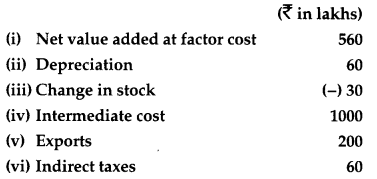Sales = Net value added at factor cost + Depreciation + Intermediate cost + Indirect taxes – Changes in stock
= (i) + (ii) + (iv) + (vi) – (iii)
= 560 + 60 + 1000 + 60 – (-30) = ₹ 1710 lakhs

Question 27.
Giving reasons categorise the following into stock and flow: 
(i) Capital
(ii) Saving
(iii) Gross domestic product
(iv) Wealth
OR
Explain the circular flow of income.
(i) Capital is a stock. This is so because capital is measured at a point of time.
(ii) Saving is a flow. This is so because it relates to a period of time.
(iii) Gross domestic product is a flow because it relates to a time period which is generally one fiscal year.
(iv) Wealth is a stock because it is measured at a point time.
OR
The flow of production, income generation and expenditure involving different sectors of the economy in the form of wages, rent and dividends, is known as circular flow of income (see diagram). Production gives rise to income (factor incomes), which in turn gives rise to demand for good and services. This demand leads to expenditure by households on goods and services produced. In this way income generated by production units reaches back to production units and makes the circular flow complete.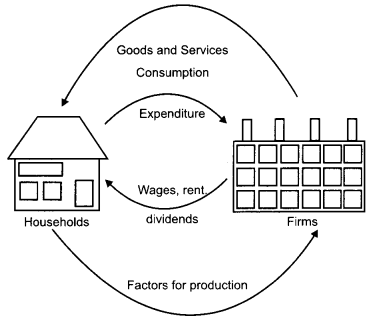Question 28.
Explain Banker to the Government function of the central bank. 
The central bank works as a commercial bank for the government. Central bank provides all services and facilities to the government like ordinary banks provide services to . public. Central bank manages all government departments undertaking and the funds of government. It provides loans to government when expenditure exceeds the revenue. This is called deficit financing through borrowing from RBI. It manages public debt also. It also accepts the payment of taxes from the public on behalf of the government and makes payment for the cheques issued by government.

Question 29.
C = 100 + 0.4 Y is the Consumption Function of an economy where C is Consumption Expenditure and Y is National Income. Investment expenditure is 1100. Calculate 
(i) Equilibrium level of National Income.
(ii) Consumption expenditure at equilibrium level of National Income.
Given, Y = National Income, Consumption Function, C = 100 + 0.4Y, Investment Expenditure, I = 1,100
(i) Now, Y = C + I
∴ Y = 100 + 0.4Y + 1,100 or y – 0.4y = 1200
or 0.6 Y = 1,200
∴ Equilibrium level of National Income,
Y = 1,200 × $$\frac{10}{6}$$ = 2,000
(ii) Now, C = 100 + 0.4Y or C = 100 + 0.4(2,000)
Consumption, C = 100 + 800 = 900

Question 30.
Complete the following table :Question 31.
Calculate National Income from the following data: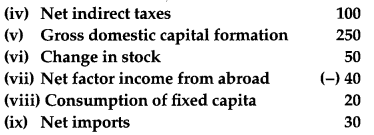OR
Calculate net national disposable income from the following data :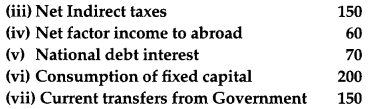National Income = Private final consumption expenditure + Government final consumption expenditure + Gross domestic capital formation + Net factor income from abroad – Consumption of fixed capital – Net imports – Net indirect taxes
= (i) + (iii) + (v) + (vii) – (viii) – (ix) – (iv)
= 900 + 400 + 250 + (-40) – 20 – 30 – 100 = ₹ 1,360 Crores

### CBSE Previous Year Question Papers Class 12 Economics 2013 Delhi Set – II

Note : Except for the following questions, all the remaining questions have been asked in previous sets.

Section – A

Question 1.
Give two examples of variable costs. 

• Wages of casual labourers who work on daily wage rate
• Cost of raw material.

Question 8.
A firm’s revenue rises from ₹ 400 to ₹ 500 when the price of its product rises from ₹ 20 per unit to ₹ 25 per unit. Calculate the price elasticity of supply. 
Initial Total Revenue (TR1) = ₹ 400
Final Total Revenue (TR2) = ₹ 500
Initial Price (P1) = ₹ 20
Initial Price (P2) = ₹ 25
⇒ Change in Price (ΔP) = ₹ (25 – 20) = ₹ 5
Initial Quantity Supplied (Q2)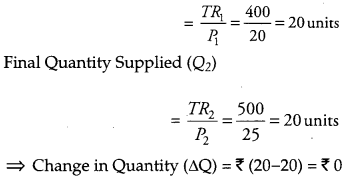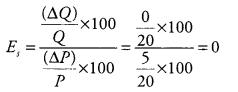Hence, elasticity of supply is zero.

Question 9.
Complete the following table.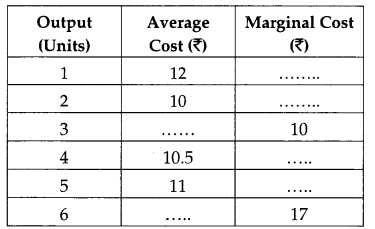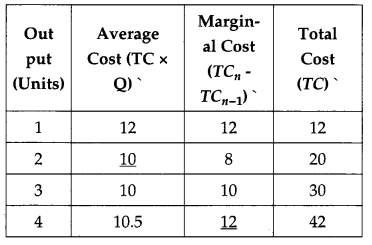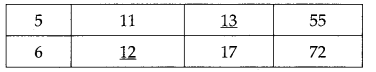Question 10.
Explain any two features of monopoly market. 
The following are the two features of a monopoly market.
(i) Restricted entry of new firms: The entry into the monopolist market is restricted. In other words, no new firm can enter the monopoly market. There may be various legal barriers such as, patent rights, cartel laws, exclusive rights, etc., to restrict the entry of the new firms.

(ii) There is only one single seller: Since, a monopolist firm is the single firm in the market, therefore, it enjoys full control over the price and output decisions. The monopolist has the total freedom to fix the price level, which maximizes his profit. Therefore, it can be said that a monopoly firm is a price-maker.

(iii) There are no close substitutes: There is no competition in the market as there is no close substitute for the product sold by monopolist.

Question 12.
The demand for good rises by 20 percent as a result of fall in its price. Its price elasticity of demand (-) 0.8. Calculate the percentage fall in price. 
OR
How is price elasticity of demand affected by:
(i) Number of substitutes of available for the good.
(ii) Nature of the good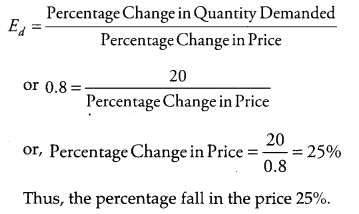OR
(i) Number of Substitutes Available for the Good: The demand for a good that has more number of substitutes available will be relatively more elastic and ed > 1. This is because a slight increase in the price will push the consumers to shift their demand away from the good to its substitutes. On the other hand, with a slight fall in price the consumers would shift their demand from the substitutes towards the good. Thus, the goods having a large number of close substitutes will have elastic demand. On the contrary, if a good has no close substitutes, then it will have an inelastic demand.

(ii) Nature of the good: The price elasticity of demand depends on the nature of a good. The goods and services can be broadly divided into three categories – Necessities, Luxuries, Jointly- demanded goods. The three types of goods have different values of elasticity as discussed below.

(a) Necessity goods: These goods are those goods which a consumer demands for sustaining his life. A consumer cannot reduce the consumption of these goods. The demand for such goods does not change much in response to the changes in their prices. Even when the price rises the consumer cannot reduce their demand. Hence, such goods have an inelastic demand (ed < 1).

(b) Luxury goods: Luxuries are the good which are not essential, rather, are consumed for leisure or comfort purposes. For example, air conditioner, branded garments, etc. The demand for such goods is highly responsive to changes in their prices. A rise in the price, reduces the demand for them and vice-versa. Thus, such goods have high price elasticity.

(c) Jointly-demanded goods: Jointly-demanded goods are those goods that are demanded together. The joint consumption of such goods collectively satisfies wants. For example, sugar and tea. A rise in the price of one good does not reduce its demand if the demand for its complement good has not reduced. For example, a rise in the price of sugar will not reduce its demand if the demand for tea has not decreased. Hence, such goods have an inelastic demand (ed <1).

Section – B

Question 28.
How do commercial banks create deposits? Explain. 
Commercial banks play an important role of ‘money creator’ in the economy. They have the capacity to generate credit through demand deposits. These demand deposits make credit more than the initial deposits.

The process of money creation can be explained by taking an example of a bank XYZ. A depositor deposit ₹ 10,000 in his savings account, which will become the demand deposit of the bank. Based on the assumption that not all customers will turn up at the same day to withdraw their deposits, banks maintains a minimum cash reserve of 10% of the demand deposits, i.e., ₹ 1000. It lends the remaining amount of ₹ 9000 in the form of credit to other customers. This further creates deposits for the bank XYZ. With the cash reserve of ₹ 1000, the credit creation is worth ₹ 10,000. So, the credit multiplier is given by:
Credit multiplier = 1/CRR = 1/10% = 10
The money supply in the economy will increase by the amount (times) of credit multiplier.

Question 30.
In an economy, S = -100 + 0.6Y is the saving function, where S is saving and Y is National Income. If investment expenditure is 1100. Calculate:
(i) Equilibrium level of National Income
(ii) Consumption expenditure of equilibrium level of National Income. 
Saving function, S = -100 + 0.6 Y Investment, I = 1,100 Y = National Income
(i) We know that S = I Given S = -100 + 0.6Y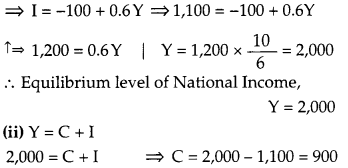∴ Consumption expenditure at equilibrium level 900

Question 32.
Complete the following table :### CBSE Previous Year Question Papers Class 12 Economics 2013 Delhi Set – III

Note: Except for the following questions, all the remaining questions have been asked in previous sets.

Section – A

Question 1.
Give an example each of fixed cost and variable cost. 
Example of Fixed Cost – Rent, Interest etc.
Example of Variable Cost – Expenditure on raw material etc.

Question 5.
The price elasticity of supply of a good is 0.8. Its price rises by 50 percent. Calculate the percentage increase in its supply. 
es = 0.8
DP = 50%
DQ = ?Percentage Change in Quantity Supplied is 40%.

Question 9.
Complete the following table :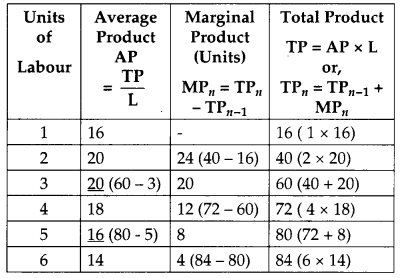Question 10.
Explain “freedom of entry and exit to firms in industry” features of monopolistic competition. 
Freedom of entry and exit to firms in a monopolistic industry implies that there exists no restriction for the new entrants and existing firms to quit the industry. The new firms are attracted into the industry whenever price exceeds the minimum of short run average cost (SAC) in short run and whenever price exceeds the minimum of long run average cost (LAC) in the long run. Due to the free entry of the new firms in the industry, the quantity produced increases, which results in the rise of the supply of the output and finally, this pushes the equilibrium price down. Thus, the equilibrium price continues to fall, until it become equals to the minimum of average cost curve, where, all the firms earns normal profit.

At this point, no new firm has any incentive to enter the industry. Similarly, on the other hand, the existing firms leave the industry whenever the price falls short of the minimum of short run average cost curve (SAC) in the short run or whenever price falls short of the minimum of long run average cost (LAC) in the long run. This is because under such circumstances, the existing firms each losses and consequently wishes to quit. Now, as there are no restrictions imposed on the exit of the firms, so firm quits the industry. This leads to the fall in the supply of the commodity. Hence, the price start rising and reaches the point at LAC where a firm earns only normal profit.

Thus, it is due to freedom of the entry and exit to firms that price remains equal to the minimum of average cost implying the normal profit only.

Question 12.
Give the meaning of producer’s equilibrium. A produces that quantity of his product at which marginal cost and marginal revenue are equal. Is he earning maximum profits? Give reasons for your answer. 
At a point where, MC = MR, the producer’s equilibrium is established. Yes, the producer earns maximum profit on this point. Because any deviation from this position will either reduce the profit of a firm or increase the losses.

MC refers to the additional cost that takes place by producing an additional unit of output. MR refers to the additional revenue that takes place by selling an additional unit.

When marginal cost is less than MR available from the sale of a product, the firm will go on increasing its output. When MC and MR are equal, the firm reaches on its equilibrium. After that point if a firm increases its output, MC will be greater than MR resulting in decline in profit. This diagram is related to the perfect competition, where the equilibrium output is OQ, where MR = MC.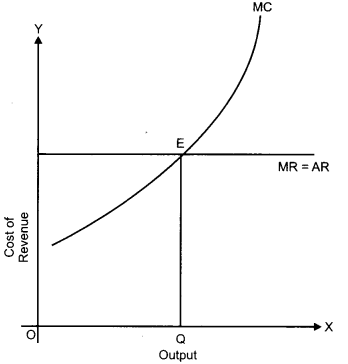Section – B

Question 27.
Calculate “sales” from the following data: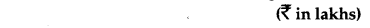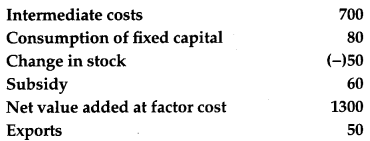NVAFC = ₹ 1,300
GDPMP = NVAFC – Subsidies + Consumption of fixed capital
= 1,300 – 60 + 80 = ₹ 1,320
Also, we know that:
GDPMP = Sales + Change in stock – Intermediate Cost
Sales = GDPMP – Change in stock + Intermediate Cost
= 1,320 – (-50) + 700
i.e. Sales = ₹ 2,070 Lakhs.

Question 31.
C = 50 + 0.5Y is the consumption function where C is consumption expenditure and Y is National Income and investment expenditure is 2,000 in an economy.
Calculate (i) Equilibrium level of (National Income) (ii) Consumption expenditure at equilibrium level. 
Y = National Income, Consumption Function, C = 50 + 0.5Y Investment,
I = 2,000
(i) Y = C + I or Y = 50 + 0.5Y + 2,000
or 0.5Y = 50 + 2,000 = 2,050or Y = 2,050 × $$\frac{10}{5}$$ = 4,100
Equilibrium level of National Income = 4,100

(ii) Given, C = 50 + 0.5Y
C = 50 + 0.5 (4,100)
C = 50 + 2,050 = 2,100
Consumption expenditure at equilibrium level = 2,100

Question 32.
Complete the following table :